# CBSEtips.in

## Saturday, 20 February 2021

### CBSE Class 9 Maths - MCQ and Online Tests - Unit 6 - Lines and Angles

#### CBSE Class 9 Maths – MCQ and Online Tests – Unit 6 – Lines and Angles

Every year CBSE  schools conducts Annual Assessment exams for 6,7,8,9,11th standards. These exams are very competitive to all the students. So our website provides online tests for all the 6,7,8,9,11th standard’s subjects. These tests are also very effective and useful for those who preparing for any competitive exams like Olympiad etc. It can boost their preparation level and confidence level by attempting these chapter wise online tests.

These online tests are based on latest CBSE syllabus. While attempting these our students can identify the weak lessons and continuously practice those lessons for attaining high marks. It also helps to revise the NCERT textbooks thoroughly.

#### CBSE Class 9 Maths – MCQ and Online Tests – Unit 6 – Lines and Angles

Question 1.
An exterior angle of a triangle is 105° and its two interior opposite angles are equal. Each of these equal angles is
(a) 37 $$\frac{1°}{2}$$
(b) 52 $$\frac{1°}{2}$$
(c) 72 $$\frac{1°}{2}$$
(d) 75°

Answer: (b) 52 $$\frac{1°}{2}$$

Question 2.
Each angle of an equilateral triangle is
(a) 50°
(b) 90°
(c) 54°
(d) 60°

Question 3.
In figure if ?A + ?B + ?C + ?D + ?E + ?F = k right angles, then find value of k.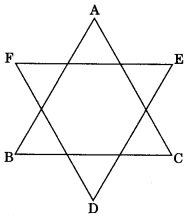(?) 2
(b) 3
(c) 4
(d) 5

Question 4.
In figure, find the value of y.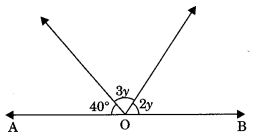(a) 28°
(b) 40°
(c) 140°
(d) 56°

Question 5.
In the given figure, the measure of ?ABC is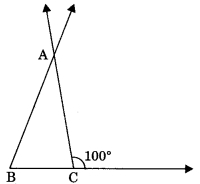(a) 80°
(b) 20°
(c) 100°
(d) 60°

Question 6.
The angle of a triangle are in the ratio 5 : 3 : 7, the triangle is
(?) an acute-angled triangle
(b) an obtuse angled triangle
(c) an right angled triangle
(d) an isosceles triangle.

Answer: (?) an acute-angled triangle

Question 7.
In figure l1 || l2, the value of x is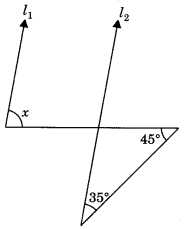(a) 80°
(b) 100°
(c) 110°
(d) 70°

Question 8.
If one angle of triangle is equal to the sum of the other two, then the triangle is
(a) an isosceles triangle
(b) an obtuse-angled triangle
(c) an equilateral triangle
(d) a right triangle

Answer: (d) a right triangle

Question 9.
One of the angles of a triangle is 75°. If the difference of other two is 35°, then the largest angle of other two angles has a measure
(a) 80°
(b) 75°
(c) 70°
(d) 135°

Question 10.
In figure the value of x is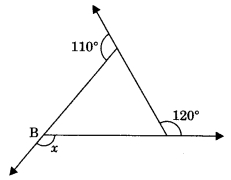(a) 120°
(b) 130°
(c) 110°
(d) 100°

Question 11.
In Figure measure of ?ABC is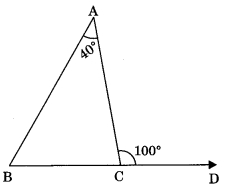(a) 60°
(b) 70°
(c) 80°
(d) 50°

Question 12.
In Figure AB || CD, the value of x is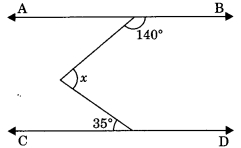(a) 35°
(b) 40°
(c) 60°
(d) 75°

Question 13.
In the figure, PS ? l, RQ ? l, the degree measure of y is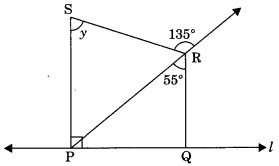(a) 55°
(b) 90°
(c) 80°
(d) 135°

Question 14.
An exterior angle of a triangle is 80° and the interior opposite angles are in the ratio 1 : 3, measure of interior opposite angles are
(a) 30°, 90°
(b) 40°, 120°
(c) 20°, 60°
(d) 30°, 60°

Answer: (c) 20°, 60°

Question 15.
Given lines l1 || l2 and l2 || l3 in figure. The value of x is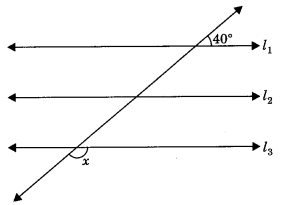(a) 40°
(b) 140°
(c) 50°
(d) 80°

Question 16.
In ?ABC, the bisectors of ?ABC and ?BCA intersect each other at O. The measure of ?BOC is
(a) 90° + ?A
(b) 90° + $$\frac{?A}{2}$$
(c) 180 – ?A
(d) 90° – $$\frac{?A}{2}$$

Answer: (b) 90° + $$\frac{?A}{2}$$

Share: